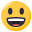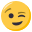# Infixa to pos-fixa

• Hi people,
I need to hand in a lesson in C language that change an infixa expression to pos-fixa.
Please, if anyone has a sorce give it to me!
it is like that:
infixa = (A+BC)
posfixa = ABC
+

***********

infixa = (A*(B+(C*(D+(E*(F+G))))))
postfixa = ABCDEFG+++*

thank u.
bye

• Dieser Thread wurde von Moderator/in evilissimo aus dem Forum C++ in das Forum ANSI C verschoben.

Im Zweifelsfall bitte auch folgende Hinweise beachten:
C/C++ Forum :: FAQ - Sonstiges :: Wohin mit meiner Frage?

Dieses Posting wurde automatisch erzeugt.

• Firstly, you are in the wrong forum, this is C++ and not Ansi C. Secondly, we don't do any homework. If you want that someone helps you, you will have to write the code as far as you are able to and then ask questions about the specific problems you'll have.

• Please, if anyone has a sorce give it to me!

As a powerful sorcerer I strongly recommend google• i have this code:

``````main() {
char *infixaParaPosfixa;
char inf;
char *posf;
char *pi; int t; // pilha
int n, i, j;

n = strlen (inf);
posf = malloc (n * sizeof (char));
pi = malloc (n * sizeof (char));
t = 0;
pi[t++] = inf;                          // empilha '('
for (j = 0, i = 1; /*X*/ inf[i] != '\0'; ++i) {
// a pilha está em pi[0..t-1]
switch (inf[i]) {
char x;
case '(': push[t++] = inf[i];          // empilha
break;
case ')': while (1) {                // desempilha
x = pop[--t];
if (x == '(') break;
posf[j++] = x;
}
break;
case '+':
case '-': while (1) {
x = pi[t-1];
if (x == '(') break;
--t;                    // desempilha
posf[j++] = x;
}
pi[t++] = inf[i];          // empilha
break;
case '*':
case '/': while (1) {
x = pi[t-1];
if (x == '(' || x == '+' || x == '-')
break;
--t;
posf[j++] = x;
}
pi[t++] = inf[i];
break;
default:  posf[j++] = inf[i];
}
}
free (pi);
posf[j] = '\0';
return posf;
}
}
``````

but it don't work

• Do you get any errors?

• i think your problem is that you're trying to return a char pointer from main, i'm talking about posf. how about you use printf to get the string pointed to on your screen? and by the way "omfgnoworky" isn't an error description.

edit: returning a pointer to a allocated resources will cause memory leaks if you forget to delete them properly. you should try to avoid this...